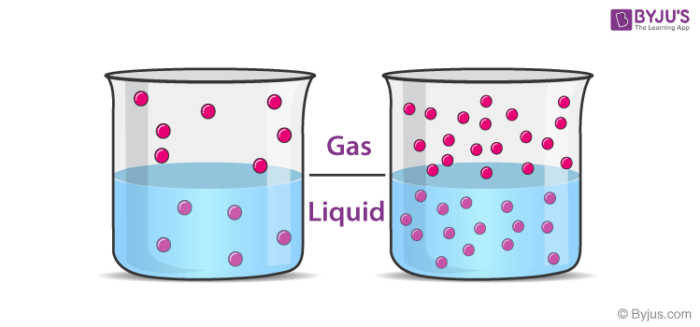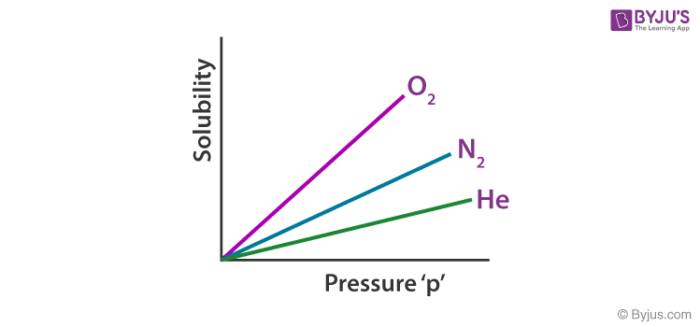# Henry's Law

## What is Henry’s Law?

Henry’s law is a gas law which states that at the amount of gas that is dissolved in a liquid is directly proportional to the partial pressure of that gas above the liquid when the temperature is kept constant. The constant of proportionality for this relationship is called Henry’s law constant (usually denoted by ‘kH‘). The mathematical formula of Henry’s law is given by:

P ∝ C (or) P = kH.C

Where,

• ‘P’ denotes the partial pressure of the gas in the atmosphere above the liquid.
• ‘C’ denotes the concentration of the dissolved gas.
• ‘kH’ is the Henry’s law constant of the gas.

## Introduction

This law was formulated in the early 19th century by the English chemist William Henry. It can be noted that the Henry’s law constant can be expressed in two different ways. If the constant is defined in terms of solubility/pressure, it is referred to as the Henry’s law solubility constant (denoted by ‘H’). On the other hand, if the proportionality constant is defined in terms of pressure/solubility, it is called the Henry’s law volatility constant (denoted by ‘kH’).An illustration detailing the relationship between the solubility of a gas in a liquid and the partial pressure of that gas in the atmosphere above the liquid (as dictated by Henry’s law) is provided above. Note that the greater the partial pressure of the gas, the greater its solubility in the liquid.

## Examples of Henry’s Law

### Pepsi and other Carbonated Drinks

Henry’s law comes into play every time a bottle of Pepsi (or any other carbonated drink) is opened. The gas above the unopened carbonated drink is usually pure carbon dioxide, kept at a pressure which is slightly above the standard atmospheric pressure. As a consequence of Henry’s law, the solubility of carbon dioxide in the unopened drink is also high.

When the bottle is opened, the pressurized CO2 escapes into the atmosphere (which is usually accompanied by a hissing sound). As the partial pressure of CO2 in the atmosphere above the drink rapidly decreases, the solubility of the carbon dioxide in the drink also decreases (due to Henry’s law). This causes the dissolved CO2 to come to the surface of the drink in the form of tiny bubbles and escape into the atmosphere.

If the carbonated drink is left open long enough, the concentration of carbon dioxide in the drink will reach an equilibrium with the concentration of carbon dioxide in the atmosphere (~0.05%), causing it to go flat (the drink loses its ‘fizzy’ taste).

### Respiration and the Oxygenation of Blood

In the process of respiration, inhalation is accompanied by an increase in the partial pressure of oxygen in the alveoli. When deoxygenated blood interacts with the oxygen-rich air in the alveoli, the following gas-exchanges take place as a consequence of Henry’s law:

• Since the partial pressure of oxygen in the alveoli is high and the amount of dissolved oxygen in the deoxygenated blood is low, oxygen flows from the alveoli into the deoxygenated blood.
• The partial pressure of carbon dioxide in the alveoli is very low (CO2 constitutes approximately 0.05% of the atmosphere). Since the concentration of dissolved CO2 in the deoxygenated blood is very high, the gas moves from the blood into the alveoli. This carbon dioxide is expelled from the body via exhalation.

Thus, Henry’s law plays an integral role in the respiration of many organisms.

## Recommended Videos## Factors Affecting the Henry’s Law Constant

The value of the Henry’s law constant of a gas is dependent on the following factors:

• The nature of the gas
• The nature of the solvent
• Temperature & pressure

Therefore, different gases have different Henry’s laws constant in different solvents, as illustrated graphically below.## Limitations of Henry’s Law

• This law is only applicable when the molecules of the system are in a state of equilibrium.
• Henry’s law does not hold true when gases are placed under extremely high pressure.
• The law is not applicable when the gas and the solution participate in chemical reactions with each other.

## Solved Examples

### Example 1

Calculate the solubility of gaseous oxygen in water at a temperature of 293 K when the partial pressure exerted by O2 is 1 bar. (Given: kH for O2 34840 bar.L.mol-1)

As per Henry’s law, P = kH*C

Substituting, kH = 34840 bar.L.mol-1 and P = 1 bar, the equation becomes

C = 1/34840 mol.L-1 = 2.87*10-5 mol/L

Therefore, the solubility of oxygen in water under the given conditions is 2.87*10-5 M.

### Example 2

The value of kH for carbon dioxide at a temperature of 293 K is 1.6*103 atm.L.mol-1. At what partial pressure would the gas have a solubility (in water) of 2*10-5 M?

Substituting the given values kH = 1.6*103 atm.L.mol-1 and C = 2*10-5 M into the Henry’s law formula:

P = kH*C = (1.6*103 atm.L.mol-1) * (2*10-5 mol.L-1) = 0.032 atm.

## Frequently Asked Questions on Henry’s Law

Q1

### Henry’s Law does not apply to which gas? Why?

• Gases such as NH3 and CO2 do not obey Henry’s law.
• This is due to the fact that these gases react with water.
NH3 +H2O → NH4+ + OH
CO2 + H2O → H2CO3
• They have higher solubilities than expected by Henry’s law due to reactions of gases such as NH3, and CO2(g).
Q2

### What are the conditions for using Henry’s Law?

Henry’s law is only applicable when the molecules are in equilibrium. Henry’s law does not apply to gases at high pressures (for example, N2(g) at high pressure becomes very soluble and dangerous when introduced into the blood supply).

Q3

### What does Henry’s law constant depend on?

It’s important to remember that Henry’s law constants are highly temperature-dependent because vapour pressure and solubility are both temperature-dependent.

Q4

### What is the unit for Henry’s law constant?

Henry’s law constant is expressed in mol L–1 bar–1.

Q5

### What are the Limitations of Henry’s Law?

The limitations of Henry’s law are as follows:

• The law is only valid when the molecules in the system are in equilibrium.
• When the gases are under extremely high pressure, Henry’s Law does not apply.
• This law also does not apply when the solution and gas are involved in a chemical reaction with each other.